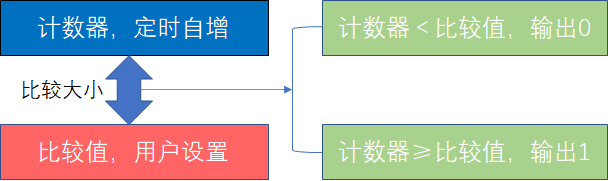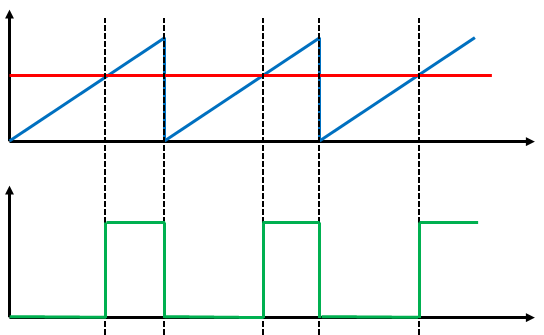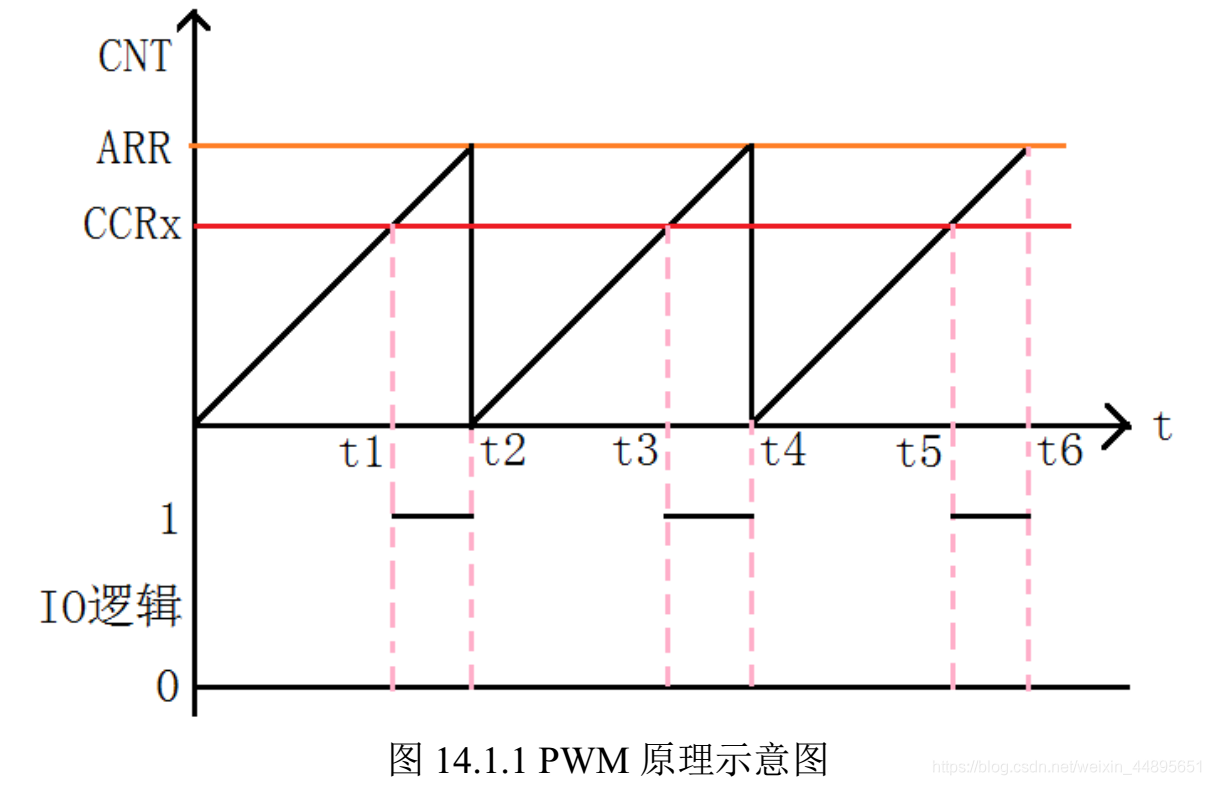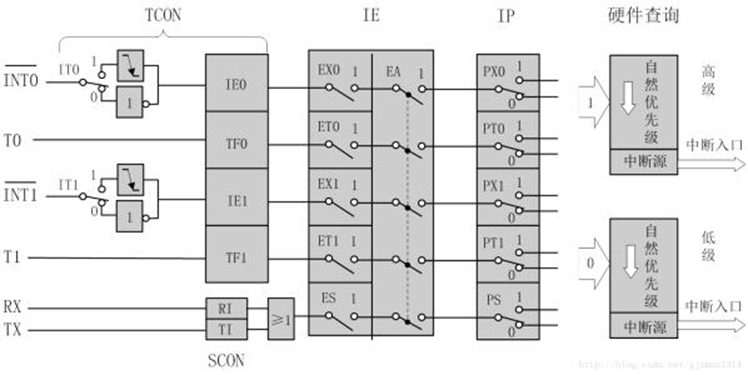# 前言

#新人报道我是普通本科大一新生，因为兴趣爱好加入学校机器人协会，计划本学期挑战电赛|ू･ω･` )没想到疫情突发，只能匆匆用了一个星期入门51，做阶段性小项目，基于51智能红外寻迹小车，虽然论坛教程很多，但还是发此贴记录分享撸车的时光，也顺便做个引子给刚入门的同好们…

# 一、准备工作

1.安装keil 5
2.有一定的c语言基础
3.明白各模块工作原理，才能写逻辑

# 二、使用步骤

## 1.模块化编程

【视频教程：b站江科大自化协】我跟着这位老师入门的51，他讲的很好，其中教会了我模块化编程（可以有效降低代码耦合性，并且写下来思路非常清晰！！！），所以在接下的说明中我会按照这种方式来讲解代码(￣▽￣)~*
（代码不成熟，仅供参考）

## 2.电机模块

``````#include <REGX52.H>

sbit IN1 = P1^0;//左轮
sbit IN2 = P1^1;//左轮
sbit IN3 = P1^2;//右轮
sbit IN4 = P1^3;//右轮

void Left_Motor_Forward()//左轮前进
{
IN1 = 1;
IN2 = 0;
}

void Right_Motor_Forward()//右轮前进
{
IN3 = 1;
IN4 = 0;
}
//
void Left_Motor_Back()//左轮后退
{
IN1 = 0;
IN2 = 1;
}

void Right_Motor_Back()//右轮后退
{
IN3 = 0;
IN4 = 1;
}
//
void Left_Motor_Stop()//左轮停
{
IN1 = 0;
IN2 = 0;
}

void Right_Motor_Stop()//右轮停
{
IN3 = 0;
IN4 = 0;
}
``````

``````#ifndef __MOTORSET_H__
#define __MOTORSET_H__

void Left_Motor_Forward();
void Right_Motor_Forward();
void Left_Motor_Back();
void Right_Motor_Back();
void Left_Motor_Stop();
void Right_Motor_Stop();

#endif
``````

## 3.小车动作模块

``````#include <REGX52.H>
#include "MotorSet.h"

void Car_Go()
{Left_Motor_Forward();Right_Motor_Forward();}

void Car_Left()
{Left_Motor_Stop();Right_Motor_Forward();}

void Car_Right()
{Right_Motor_Stop();Left_Motor_Forward();}

void Car_Stop()
{Left_Motor_Stop();Right_Motor_Stop();}

void Car_Back()
{Left_Motor_Back();Right_Motor_Back();}
``````

``````#ifndef __MOTORSET_H__
#define __MOTORSET_H__

void Left_Motor_Forward();
void Right_Motor_Forward();
void Left_Motor_Back();
void Right_Motor_Back();
void Left_Motor_Stop();
void Right_Motor_Stop();

#endif
``````

## 4**.PWM**和定时器、中断系统CSDN上也有足够详细的讲解：
pwm的频率：

pwm的周期：
T=1/f

50Hz = 20ms 一个周期（图片为定时器和中断系统的原理图）

``````#include <REGX52.H>

sbit ENA_1 = P3^0;
sbit ENA_2 = P3^1;
sbit ENB_1 = P3^2;
sbit ENB_2 = P3^3;

/**
* @brief  定时器0初始化，100us@12.000MHz
* @param  无
* @retval 无
*/
void Timer0_Init(void)		//100微秒@11.0592MHz
{

TMOD &= 0xF0;		//设置定时器模式
TMOD |= 0x01;		//设置定时器模式
TL0 = (65536-100)%256;		//设置定时初始值
TH0 = (65536-100)/256;		//设置定时初始值
TF0 = 0;		//清除TF0标志
TR0 = 1;		//定时器0开始计时
ET0 = 1;
EA = 1;
PT0 = 0;
}

``````

``````
unsigned int pwml,pwmr,t;//左右占空比，比较值
void Timer0_Routine() interrupt 1
{
TL0 = (65536-100)%256;		//设置定时初始值
TH0 = (65536-100)/256;		//设置定时初始值
t++;
if(t<pwml)
{
ENA_1 = 1;
ENA_2 = 1;
}
else
{
ENA_1 = 0;
ENA_2 = 0;
}
//左pwm
if(t<pwmr)
{
ENB_1 = 1;
ENB_2 = 1;
}
else
{
ENB_1 = 0;
ENB_2 = 0;
}
//右pwm
if(t>=100){t = 0;}
}

``````

``````#ifndef __TIMER0_H__
#define __TIMER0_H__

extern pwml;
extern pwmr;
extern t;
void Timer0_Init(void);

#endif

``````

## 5.寻迹逻辑

``````#include <REGX52.H>
#include "CarGo.h"
#include "Timer0.h"
#include "Delay.h"

sbit OUT1 = P2^5;//左大调
sbit OUT2 = P2^4;//左微调
sbit OUT4 = P2^2;//右大调
sbit OUT3 = P2^1;//右微调

sbit IN1 = P1^0;//左轮
sbit IN2 = P1^1;//左轮
sbit IN3 = P1^2;//右轮
sbit IN4 = P1^3;//右轮

extern pwml;
extern pwmr;

void Chack()
{
if(OUT1 == 1 && OUT2 == 1 && OUT3 == 1 && OUT4 == 1)//全黑线（或空中）停车
{
pwml = 0;
pwmr = 0;
Car_Stop();
}

if(OUT1 == 0 && OUT2 == 0 && OUT3 == 1 && OUT4 == 0)//偏左，右移
{
pwml = 20;
pwmr = 16;
Car_Go();
}

if(OUT1 == 0 && OUT2 == 1 && OUT3 == 0 && OUT4 == 0)//偏右，左移
{
pwml = 16;
pwmr = 20;
Car_Go();
}
///
if(OUT1 == 1 && OUT2 == 1 && OUT3 == 0 && OUT4 == 0)//左大转
{
//		Car_Stop();
//		Delay(20);
//		pwml = 5;
//		pwmr = 30;
//		Delay(20);
//		Car_Go();

while(1)
{
IN1 = 0;
IN2 = 1;
if(OUT3 == 1) {
IN1 = 1;
IN2 = 0;
break;}
pwml = 18;
pwmr = 18;
Car_Go();

}
}
//	if(OUT1 == 0 && OUT2 == 0 && OUT3 == 0 && OUT4 == 1)//右大转
//	{
Car_Stop();
Delay(20);
pwml = 5;
pwmr = 30;
Delay(20);
Car_Go();
//
//		while(1)
//		{
//			IN3 = 0;
//				IN4 = 1;
//				if(OUT2 == 1) {
//				IN3 = 1;
//				IN4 = 0;
//				break;}
//				pwml = 25;
//				pwmr = 25;
//				Car_Go();
//
//		}
//	}
///
if(OUT1 == 1 && OUT2 == 0 && OUT3 == 0 && OUT4 == 1)
{
pwml = 16;
pwmr = 16;
Car_Go();
}
}
``````

``````#ifndef __CHACK_H__
#define __CHACK_H__
#inlcude "MotorSet.h"
void Chack();
#endif
``````